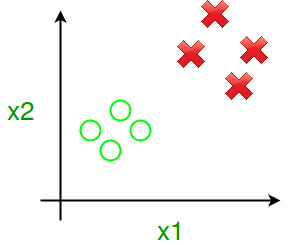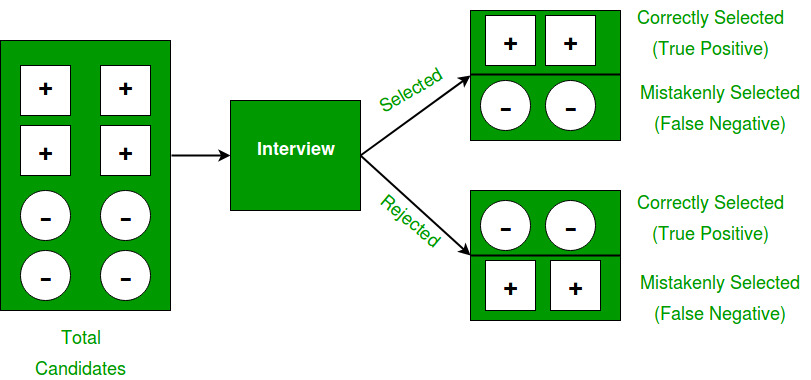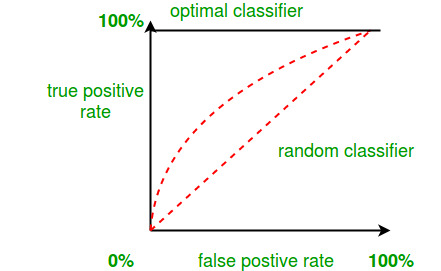Related Articles

# Calculate Efficiency Of Binary Classifier

• Last Updated : 06 Jun, 2021

Prerequisite: target=”_blank”>Getting started with Classification

In this article, we will discuss a method to calculate efficiency of Binary classifier. Let’s assume there is problem where we have to classify a product which belongs to either class A or class B.Let us define few statistical parameters :

TP (True Positive) = number of Class A products, which are classified as Class A products.
FN (False Negative) = number of Class A products, which are classified as Class B products.
TN (True Negative) = number of Class B products, which are classified as Class B products.
FP (False Positive) = number of Class B products, which are classified as Class A products.

```FP = N-TP;      // where number N is the number of class A type products
FN = M-TN;     // where number M is the number of class B type products```

We shall look at this example, to understand these parameters well.

If (+) denotes fit candidates for Job and (-) denotes unfit candidates for Job.To calculate Efficiency of classifier we need to compute values of Sensitivity, Specificity and Accuracy

Sensitivity measures the proportion of positives that are correctly identified as such.
Also known as True positive rate(TPR).

Specificity measures the proportion of negatives that are correctly identified as such.
Also known as True negative rate(TNR).

Accuracy measures how well the test predicts both TPR and TNR.

```Sensitivity = ( TP / (TP+FN) ) * 100;
Specificity = ( TN/(TN+FP) ) * 100;
Accuracy = ( (TP+TN) / (TP+TN+FP+FN) ) * 100;
Efficiency = ( Sensitivity + Specificity + Accuracy ) / 3; ```

Let’s take above example and compute efficiency of selection :

Say fit candidates belong to class A and unfit candidates belong to class B.

```Before Interview :  N = 4 and M = 4

After Interview :
TP = 2
TN = 2
FP = N - TP = 2
FN = M - TN = 2

Sensitivity = 2/(2+2)*100 = 50
Specificity = 2/(2+2)*100 = 50
Accuracy    = (2+2)/(2+2+2+2)*100 = 50
Efficiency  = (50+50+50)/3 = 50

So,Efficiency of selection of candidates is 50% accurate.```

Other performance measures:

• Error rate = (FP + FN) / (TP + TN + FP + FN)

• Precision = TP / (TP + FP)

• Recall = TP / (TP + FN)

• BCR (Balanced Classification Rate) = 1/2* (TP / (TP + FN) + TN / (TN + FP))

• AUC = Area under ROC curve

• Receiver operating characteristic(ROC) curve : 2-D curve parameterized by one parameter of the classification algorithm.

• AUC is always between 0 and 1.

• ROC curve can be obtained plotting TPR on y-axis and TNR on x-axis.

• AUC gives accuracy of the proposed model.References:My Personal Notes arrow_drop_up